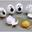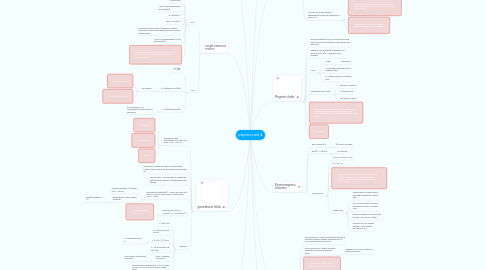# physics unit 4Helena DaCosta

AQA Pysics revision all the important information and equations with exam help in red

Get Started. It's Freephysics unit 4## 1. force and momentum

### 1.1. Momentum(p) =mxv

1.1.1. total p before = total p after

1.1.2. p is conserved in a collison but kinetic energy is not

1.1.3. Vector quality

1.1.3.1. Direction IS important

## 2. motion in a circle

### 2.4. a=v^2/r = w^2r

2.4.1. acts towards the centre of the circle. it is acceleration as the velociry is constantly changing

### 2.5. F=ma = mv^2/r = mw^2r

2.5.1. Acts towards the centre of the circle

## 3. simple harmonic motion

### 3.1. SHM

3.1.1. v=2πf(square root)A^2-x^2

3.1.1.1. vmax=2πfA

3.1.2. x=Acos(2πf)

3.1.3. f and T are unaffected by amplitude(A)

3.1.4. a=-x(2pief)^2

3.1.5. amax=-A(2πf)^2

3.1.6. Oscillations where the acceleration is always proportional and in the opposite direction to the displacement

3.1.7. f and T are independent of the amplitude(A)

3.1.8. Graphs to show one complete cycle is double what is expected left>middle>right>middle = 2 normal waves

### 3.2. SHO

3.2.1. f=-kx

3.2.2. T=2π(square root)m/k

3.2.2.1. for a spring

3.2.2.1.1. parallel k= k1+k2

3.2.2.1.2. series 1/k= 1/k1 + 1/k2

3.2.3. T=2π(square root)l/g

3.2.3.1. for a pendulum T is independent of the mass and amplitude

## 4. gravitational fields

### 4.1. graviational field strength(g)=force per unit mass= F/m = Gm/r^2

4.1.1. g= 9.8N/kg

4.1.2. G= 667x10-11

4.1.3. g=kpr

### 4.4. gravitational potential(V) = work done per unit mass to move it from infinity to that point = W/m = -Gm/r

4.4.1. potential energy=m (change in)V= -GMm/r

4.4.2. eqipotential= lines of equal potential

### 4.5. gravitational force F = -GMm/r^2 =- GMm/(R+r)^2

4.5.1. F is proportional to 1/r^2

### 4.6. Satelites

4.6.1. v^2=gR^2/h

4.6.2. v= (square root of) 2GM/R

4.6.3. T^2=4(π^2)r^3/GM

4.6.3.1. T^2 is proportional to r^3

4.6.4. T= 2π/R (square root of)r^3/g

4.6.5. Polar = weather forcasting

4.6.5.1. Ek increases as potential decreases

4.6.6. geosynchronous/stationary = tv as it takes 24h for 1 cycle so it stays above a fixed point

## 5. Electric fields

### 5.3. uniform field- produced by connecting two plates to opposite sides of a battery. The current flows from + to -

5.3.1. Electric field strength(E)=force per unit charge=F/Q

5.3.1.1. E=V/d for a uniform field

5.3.2. E is the same at all points in a uniform field

5.4.1. F=(1/4πeo) Qq/r^2

5.4.1.1. 1/4πeo = 9x10+9

5.4.1.2. E=(1/4πeo) Q/r^2

5.4.1.2.1. E=V/r

5.4.1.3. V=(1/4πeo) Q/r

5.4.2. For a point charge the field lines can point towards the particle(-ve charge) or away from the particle(+ve charge)

## 6. Capacitors

### 6.1. capitance(C)=Q/V=charge stored per volt=It

6.1.1. Q=Qoe^(-t/RC)

6.1.2. this equation is true for Q, V, I

### 6.4. t=CR= time taken to charge to 63% of its total or discharge to 37% total. After 5 t the capacitor is considered fully charges/discharged

6.4.1. t=-ln(Q/Qo)RC

### 6.6. You can use a data logger in experiments to find the capacitance using C=It

6.6.1. Disadvantages: it has a low resistance of 40k ohms so if the resistance in the circuit is equal it will not work.

6.6.2. Advantages: it can record for small intervals and take lots of readings.

## 7. Magnetic fields

### 7.3. F=BIL

7.3.1. F=Bqv

7.3.1.1. F=BQ/2mπ

7.3.2. F=max when perpendicular to magnetic field

7.3.3. F=0 when parallel to magnetic field

### 7.4. Flemings left hand rule

7.4.1. thumb=movement

7.4.2. 1st finger=field

7.4.3. 2nd finger=current

## 8. Electromagnetic induction

8.1.1. ΦN=BAN.cosangle

### 8.2. Emf(ℰ) = ΔΦN/Δt

8.2.1. =Eo.sin2πft

### 8.3. transformers

8.3.1. Vs/Vp = Ns/Np = Ip/Is

8.3.2. P=I^2R= IV

8.3.3. Alternating voltage in primary coil produces an alternating magnetic field. This alternating magnetic field induces a voltage across the secondary coil.

8.3.4. Energy loss

8.3.4.1. Heating effect of the current- use high conductivity copper wire

8.3.4.2. not all the magnetic flux links with the secondary- overlap coils

8.3.4.3. energy is required to reverse the polarity- use soft iron cores

8.3.4.4. resistance in iron causes heating- use insulated, laminated cores

## 9. Resonance

### 9.2. forced vibrations = where a system vibrates by an external periodic force.

9.2.1. frequency of forced vibrations = driving frequency

### 9.4. Dampening

9.4.1. light- takes a long time to stop oscillating, reducing a small amount each period (pendulum)

9.4.1.1. sharp resonance peak

9.4.2. heavy- displaced object returns to equilibrium without oscillation.

9.4.3. critical-reduces amplitude in the shortest time possible (car suspensions

9.4.3.1. lower resonance peak

## 10. Comparing fields

### 10.1. Similarities

10.1.1. field strength= force per unit charge/mass

10.1.2. F is proportional to 1/r^2

10.1.3. both have radial fields towards on object

10.1.4. potential= potential energy per unit mass/charge

### 10.2. Differences

10.2.1. gravity is always attractive, electric can be repulsive

10.2.2. Different constants

10.2.3. Cant shield from gravity unlike electrical forces